# midas Structure

BLOG NEWS & TRENDS

OVERVIEW

The Seismic Load Analysis Procedure has 16 processes and it is largely divided into 2 parts : Series 1 (Including Series 2 , 3 & 4) and Series 5 (Modal Response Spectrum (RS) Analysis.

The part we will look over today is Series 5 : Modal Response Spectrum (RS) Analysis.

Modal Response Spectrum(RS) Analysis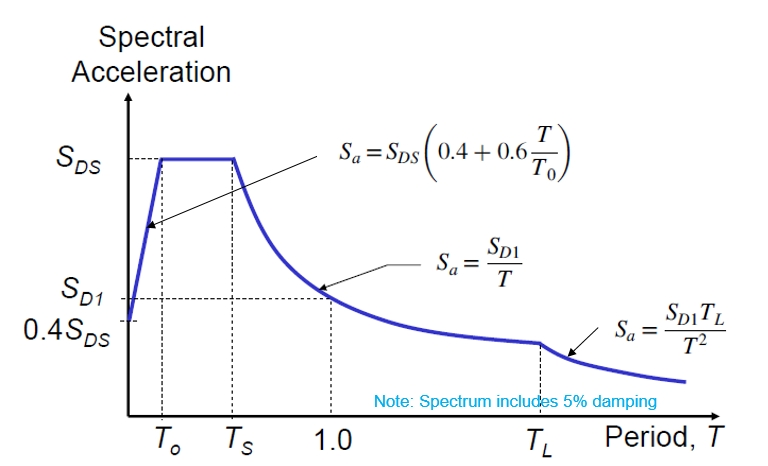Where

⋅ SDS = the design spectral response acceleration parameter at short periods

⋅ SD1 = the design spectral response acceleration parameter at a 1-sec period

⋅ T = the fundamental period of the structure, sec

⋅ T = 0.2(SD1/SDS )

⋅ TL  = long-period transition period(s) shown in Figs. 22-14 through 22-17

Basic Steps in RS Analysis

1. Compute modal properties for each mode

> Frequency (period), Shape, Modal participation factor, Effective modal mass

2. Determine number of modes to use in analysis

> Use a sufficient number of modes to capture at least 90% of total mass in each direction.

3. Compute spectral accelerations for each contributing mode

> Use a general spectrum.

4. Compute modified spectral accelerations

> Multiply spectral accelerations by modal participation factor and by (I/R).

5. Compute modal displacements for each mode

6. Compute element forces in each mode

7. Statistically combine modal displacements to determine system displacements

8. Statistically combine component forces to determine system displacements

9. Scale-up seismic load of RS

> If the design base shear based on modal analysis is less than 85% of the base shear(Equivalent Lateral force) computed using T=TaCu.

→ combination of modes must be scaled such that the base shear equals

0.85 times the equivalent lateral force.

11. Check story drift

> multiply the results of the modal analysis

(including the I/R scaling but not the 85% scaling) by Cd/I.

## 1. The Setting of RS Analysis in nGen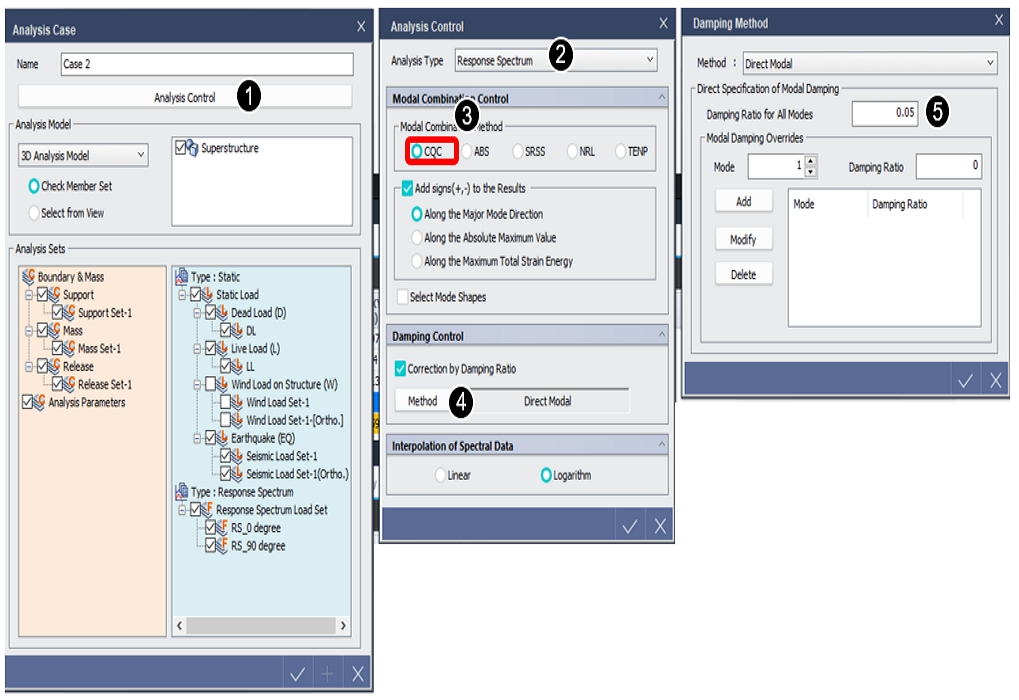1. Click "Analysis Control" in "Analysis case" dialog box.

2. Select "Response Spectrum" in analysis type.

3. Select modal combination method. (use CQC generally).

4. Click "Method" button in damping control.

5. Modify damping ratio if necessary.

## 2. Add signs(+, -) to the Results

: Specify whether to restore the signs deleted during the mode combination and specify the restoration method.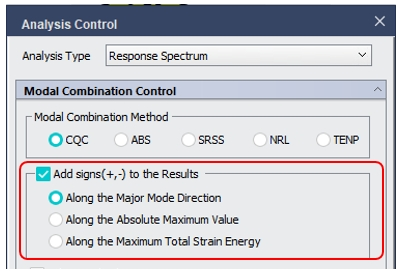Along the Major Mode Direction:  Restore the signs according to the signs(+, -) of the principal mode for every loading direction.

Along the Absolute Maximum Value: Restore the signs according to the signs of the absolute maximum values of the modal results.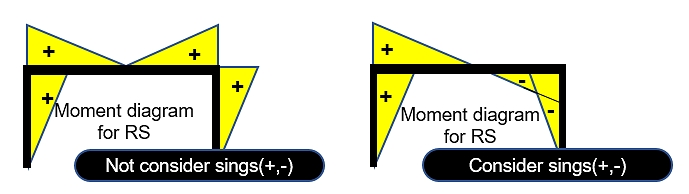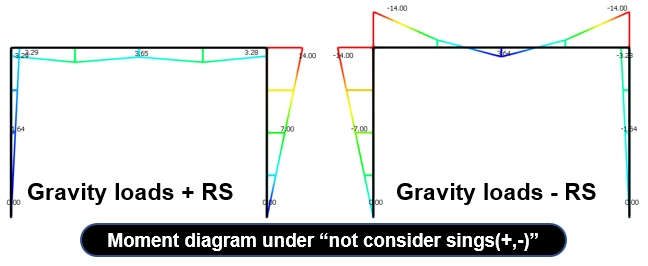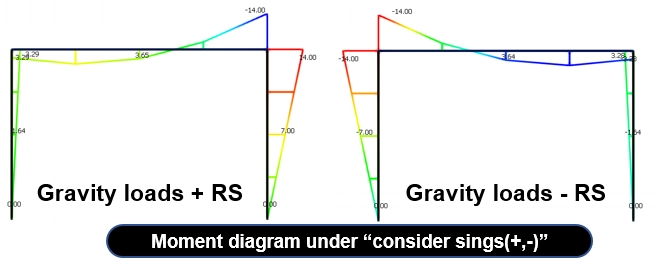In both cases, the same design results can be obtained, but the sign(+,-) must be considered in order to find the actual member force.

## 3. Analytical Modeling for RS Analysis

• Use 3D analysis
For concrete structures, include the effect of cracking (consider a stiffness for cracked section).
For steel structures, include panel zone deformations.
• Include flexibility of foundation if well enough defined
• Include actual flexibility of diaphragm if well enough defined
• Include P-delta effects in the analysis if the program has the capability
• Do not try to include accidental torsion by the movement of the center of mass
• Include orthogonal load effects by running the fill 100% spectrum in each direction, and then SRSSing the results.

## 4. Calculation of Base Shear by RS Analysis

Generation of RS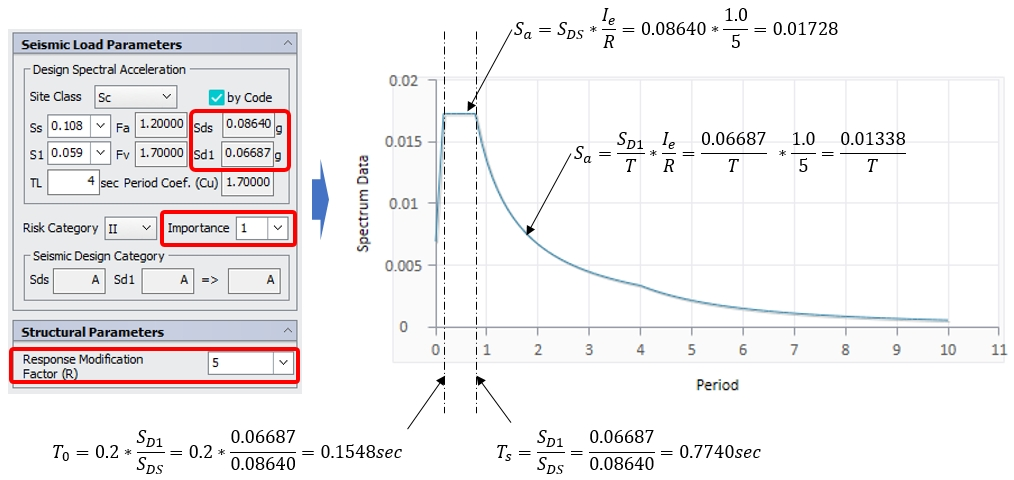Base shear(x-dir) for example model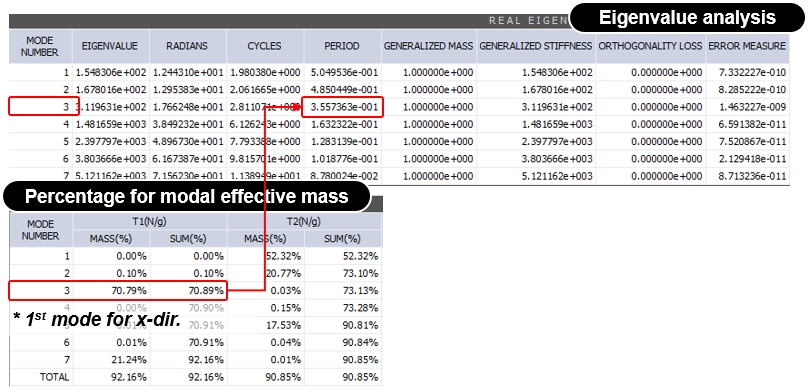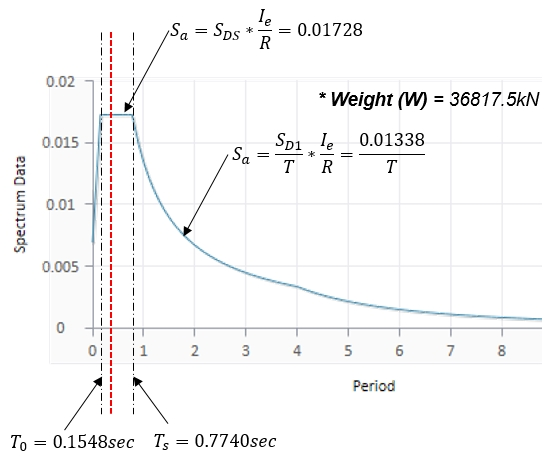𝑇=0.35574sec

So, Sa = SDS*Ie/R = 0.01728

(red dotted line)

V= W * Sa * Mass(%)

= 36817.5 * 0.01728 * 0.7089

= 451.01kN (just for 1st mode)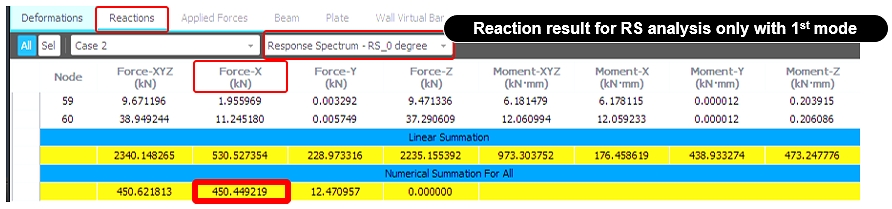Yeong-il Seo | Principal Structural Engineer

Young-il has over 13+ years of experience in building design, especially high-rise buildings with column reduction analysis, plant structures, pushover analysis, health monitoring, and vibration control projects. Since 2016, he is planning and providing technical supports for midas building products such as midas Gen, nGen, and Design+.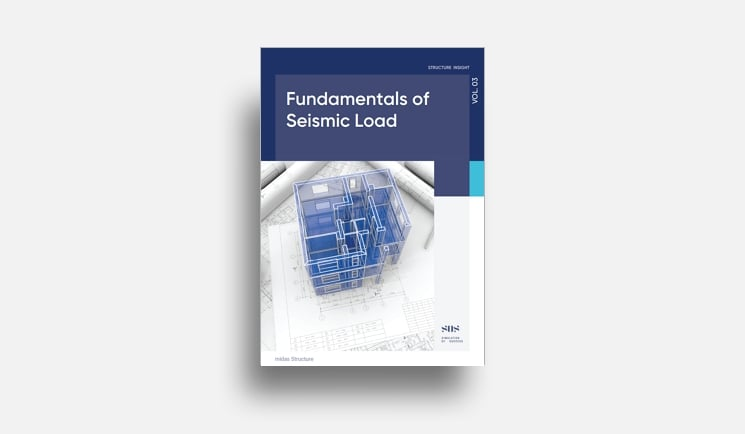E-BOOK Fundamentals of Seismic